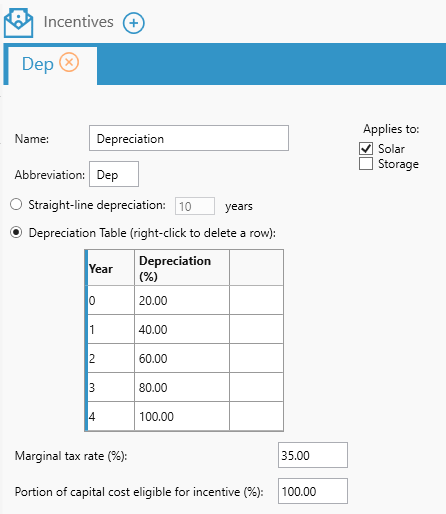HOMER Grid 1.8

 Navigation: Design > Programs > Incentives DepreciationDepreciation is a way of reducing the value of a purchased asset over its usage. It indicates how the value of the asset will decrease over time.

In HOMER Grid, you can apply a depreciation to the following components:

Solar

Storage

Below is an explanation of the various inputs in Depreciation

 Variable Description Depreciation curve (%) The year by year depreciation percentage Eligible percent (%) Portion of capital cost eligible for this incentive Marginal tax percent (%) Marginal tax rate Applies to The components that this bonus depreciation applies to

Example 1:

Let us consider a PV system of 100 kW which has a total capital cost of 100,000\$. If the capital based incentive applied to this PV system is:Depreciation in year 0 = 0.20 * Marginal Tax Rate * capital cost = 0.20 * 0.35 * 100,000\$ = 7000\$

Depreciation in year 1 = 0.40* Marginal Tax Rate * capital cost = 0.40 * 0.35 * 100,000\$ = 14,000\$

Depreciation in year 2 = 0.60* Marginal Tax Rate * capital cost = 0.60 * 0.35 * 100,000\$ = 21,000\$

Depreciation in year 3 = 0.80* Marginal Tax Rate * capital cost = 0.80 * 0.35 * 100,000\$ = 28,000\$

Depreciation in year 4 = 1 * Marginal Tax Rate * capital cost = 1 * 0.35 * 100,000\$ = 35,000\$

The Homer Support site has a searchable knowledgebase and additional support options. HOMER Online contains the latest information on model updates, as well as sample files, resource data, and contact information.UL LLC © 2022